# Absolute

(diff) ← Older revision | Latest revision (diff) | Newer revision → (diff)

The absolute of a regular topological spaceis the space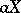which is mapped perfectly and irreducibly onto, and is such that any perfect irreducible inverse image of the spaceis homeomorphic to. Each regular spacehas a unique absolute. The absolute of a spaceis always extremally disconnected and completely regular, and is perfectly and irreducibly mapped ontoby means of a transformation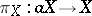. If two spacesandare connected by a single-valued or multi-valued perfect irreducible mapping, then their absolutes are homeomorphic, and there exists a homeomorphismsuch that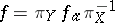.

If a homeomorphism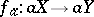is given, the mappingwill be, in the general case, multi-valued, irreducible and perfect. Thus, absolutes and their homeomorphisms "control" the entire class of perfect irreducible mappings of regular spaces. The meaning of this fundamental property is that absolutes of regular topological spaces are projective objects in the category of regular spaces and perfect irreducible mappings. If a regular spaceis compact, respectively, finally compact or complete in the sense of Čech, the respective property is also displayed by the absolute of this space. The absolute of a paracompact space is even strongly paracompact, and is, moreover, perfectly zero-dimensional. However, the absolute of a normal space need not itself be normal. Ifis a completely regular space, then the Stone–Čech compactification of its absolute is the absolute of any compactification of. Two spaces are called co-absolute if their absolutes are homeomorphic.

Thus, the class of regular spaces is subdivided into disjoint (pairwise not intersecting) classes of co-absolute spaces. A spaceis co-absolute with some metric space if and only if it is a paracompact feathered space containing a dense-discrete system of open sets. A compact space is co-absolute with some metrizable compactum if and only if it has a countable-weight. If a compact space has a countable-weight and has no isolated points, then and only then it will be co-absolute with the perfect Cantor set. Consequently, all metrizable compacta without isolated points are co-absolute with the perfect Cantor set. The absolute of a countable metrizable compactum is an extension of the Stone–Čech compactification of the natural numbers. The absolute of an extremally disconnected space is homeomorphic to it. Thus, the class of absolutes (whatever this may be) of regular spaces coincides with the class of extremally disconnected spaces. Since a non-discrete extremally disconnected space does not contain any convergent sequence of pairwise distinct points, the absolute of any non-discrete space is non-metrizable (and does not even satisfy the first axiom of countability).

Of the numerous ways in which the absoluteof a given (regular) spacecan be constructed, the following method is one of the simplest.

A familyof non-empty canonical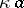-sets, i.e. of canonical closed setsof the space, is called a thread if it is inclusion-directed, i.e. if for each two elementsof the familythere exists an element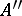contained in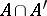. A threadis called a maximal or an end thread if it is not a subfamily of any thread different from it. It can be shown that threads exist; moreover, it can be shown that for each non-empty-setthe setof all threads containing the setas an element is non-empty. Each thread is contained in some maximal thread. The intersection of all sets which are elements of a maximal threadis either empty or contains a single point; in the latter case the threadis said to be convergent (to the point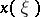). A topology is introduced in the set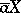of all ends (i.e. maximal threads), by taking the collection of all sets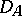as a basis for the closed sets. The resulting topology is Hausdorff and compact. The convergent ends in the compactumform an everywhere-dense subspace. The subspace of the spaceconsisting of the convergent ends is at the same time the absoluteof; it turns out thatis identical with the Stone–Čech compactification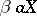of. Ifis not only regular, but completely regular, the formula of commutativity of the operators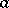andis valid: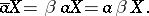The definition of the absolute of a regular topological spacegiven above is slightly imprecise. A better (more precise) definition is: The absolute of a regular topological spaceis a pair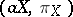, where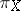is a perfect irreducible mapping ofonto, such that for every regular topological space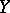and every perfect irreducible mappingofontothere is a mapping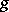ofontosuch that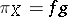.
The simple construction ofgiven above can be rephrased as follows: The family of regular closed sets forms, in a natural way, a complete Boolean algebra. The spaceis then simply the Stone space of this Boolean algebra (the set of all ultrafilters (cf. Ultrafilter) on it, topologized using the setsas a base for the closed sets).